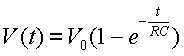# Resistive Charging / Discharging

Resistive Charging
Discharging
The formulas on this page are associated with simple circuits for both charging and discharging of a capacitor through a resistor since this can be a common model for many high voltage and pulsed power discharge circuits. Resistive charging is often used to limit the peak charging current from a constant voltage electrical source (power supply) when charging a capacitor for energy storage prior to the pulsed discharge.

Each of the following waveform plots can be clicked on to open up the full size graph in a separate window.

As will be discussed below, RC charging is simple but not very efficient (50%) in charging a capacitor. Other more efficient methods include the use of power supplies specifically designed to charge these types of loads (e.g. capacitor charging high voltage power supplies where the output current is essentially constant). As a result, the capacitor voltage rises linearly towards the target voltage.

Resistive Charging

The circuit schematic for the resistive (RC) charging case is shown below. In this specific model, the capacitor (initial condition = 0 volts) is charged from a 1000 V power supply. A 1000 ohm series resistor limits the charging current to 1 A and the switch is closed at approximately time 0. With the 1000 ohm resistor and the 1 mF capacitor, the time constant for the circuit is 1 ms.

The results of the circuit model are shown below. V(C1) is the voltage on the 1 mF capacitor as it charges up. The circuit current, I(C1), is graphed in the second, lower plot.RC Charging Circuit Equation for Voltage as a Function of Time Voltage V0 is the power supply voltage (V) C is the circuit capacitance (F) R is the circuit resistance (W)RC Charging Circuit Equation for Current as a Function of Time Current All variables defined as above

The table below shows normalized values of the voltage and current for the RC charging circuit as a function of time constant. One can see that by the time three time constants have passed, the voltage has achieved 95% of its maximum value and by the time 5 time constants have gone by, the voltage and currents have essentially reached their final values.

 Normalized Time Constant Normalized Voltage Normalized Current 0 0 1 0.105 0.1 0.9 0.223 0.2 0.8 0.357 0.3 0.7 0.511 0.4 0.6 0.693 0.5 0.5 0.916 0.6 0.4 1 0.693 0.368 1.204 0.7 0.3 1.609 0.8 0.2 2 0.865 0.135 2.302 0.9 0.1 3 0.95 0.05 4 0.982 0.018 5 0.993 0.007 10 1 0

The figure below shows the capacitor voltage, V(C1) on the top graph. The bottom graph shows the energy stored in the capacitor, ES(C1), and the energy dissipated in the charging resistor, ED(R1). One can see that by the time the capacitor is fully charged, the energy stored is equal to the energy dissipated (the charging is only 50% efficient). As noted above, because of this limitation, these circuits are frequently replaced with capacitor charging power supply systems which can be much more efficient.

RC Discharging

The circuit schematic for the RC discharge case is shown below. In this specific model, the 1 mF capacitor is initially charged to a voltage of 1000 V and the switch is closed at approximately time 0.

The results of the circuit model are shown below. V(C1) is the voltage on the 1 mF capacitor as it discharges in a simple RC decay mode. The circuit current, I(R1) through the 1000 ohm load resistor, is graphed in the second, lower plot.RC Discharge Equation for Capacitor Voltage as a Function of Time Voltage V0 is the initial voltage on the capacitor (V) C is the circuit capacitance (F) R is the circuit resistance (W)RC Discharging Circuit Equation for Current as a Function of Time Current All variables defined as above.

The table below shows normalized values of the voltage and current for the RC discharging circuit as a function of time constant. One can see that by the time three time constants have passed, the voltage and current have achieved 95% of their minimum value and by the time 5 time constants have gone by, they are essentially zero.

 Normalized Time Constant Normalized Voltage Normalized Current 0 1 1 0.105 0.9 0.9 0.223 0.8 0.8 0.357 0.7 0.7 0.511 0.6 0.6 0.693 0.5 0.5 0.916 0.4 0.4 1 0.368 0.368 1.204 0.3 0.3 1.609 0.2 0.2 2 0.135 0.135 2.302 0.1 0.1 3 0.05 0.05 4 0.018 0.018 5 0.007 0.007 10 0 0

Send consulting inquiries, comments, and suggestions to richard.ness@nessengr.com